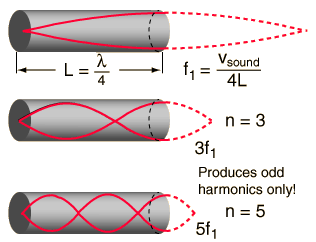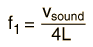# Closed Cylinder Air Column

A closed cylindrical air column will produce resonant standing waves at a fundamental frequency and at odd harmonics. The closed end is constrained to be a node of the wave and the open end is of course an antinode. This makes the fundamental mode such that the wavelength is four times the length of the air column. The constraint of the closed end prevents the column from producing the even harmonics.Calculation

The clarinet consists of an approximate closed cylinder, and this makes clarinet acoustics quite different from the other woodwind instruments. As can be seen from a sample waveform, the even harmonics missing from the tone, at least for the lower range of the instrument. The upper register of notes which may be played with this instrument start at the third harmonic of the air column rather than at the second harmonic as in the other woodwinds.

 Comparison of air columns Resonance tube experiment
Index

Wave concepts

Resonance concepts

 HyperPhysics***** Sound R Nave
Go Back

# Closed Cylinder Frequency

At temperature T = C
T = F,

the speed of sound is v= m/s
v = ft/s = mi/hr.

A closed cylindrical air column of length L = m = ft

will produce a fundamental frequency:= HzIn actual practice, the position of the antinode is slightly outside the open end, and an end correction of about 0.6 times the radius of the pipe should be added to the open end to get the effective acoustic length.

 Discussion of closed air columns Comparison of air columns Calculation for open column
 Resonance tube experiment
Index

Wave concepts

Resonance concepts

 HyperPhysics***** Sound R Nave
Go Back